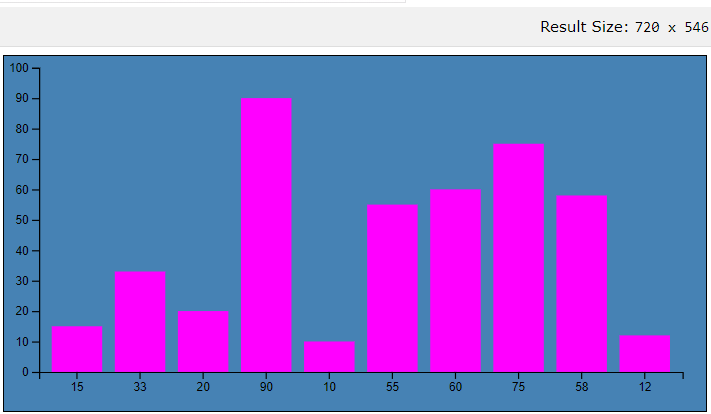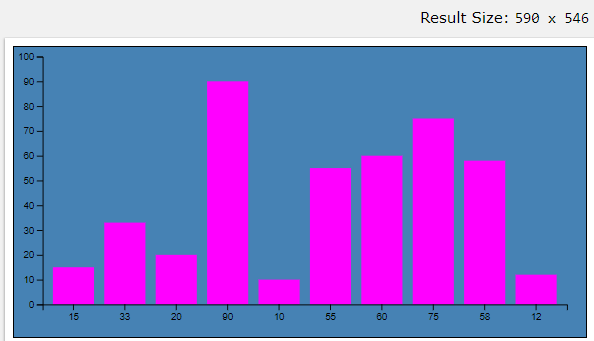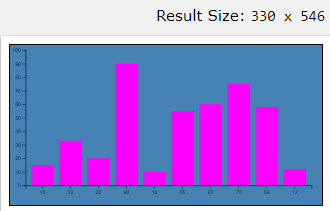# Best way to make a d3.js visualization layout responsive

D3 stands for Data-Driven Documents. It is an open-source JavaScript library that is used to create interactive data visualizations in the browser using HTML, SVG, and CSS. The huge amount of data is being generated in today’s world, which is very difficult to store and analyze. Visual representations of data are the most effective means of conveying information stored and D3 provides the ease and flexibility to create these data visualizations.

A simple way to make any SVG or D3.js chart responsive. Here we will be using D3.js to make bar graph responsive.

Approach for creating d3.js visualization responsive: Let’s look at some of the important concepts we have learned, which will be implementing through HTML code below.

• Select an element to perform operation.
`d3.select("body");`
• Use .append() method to add an element.
`var svg = d3.select("body").append("svg");`
• set.attr() is used to set the attribute (height/width)
`svg.attr({"width":500, "height":500});`
• Last step is to append the data to DOM.
```var data_values = [15, 33, 20, 90, 10, 55, 60, 75, 58, 12];

// Create rectangles
var bars = svg.selectAll("rect")
.data(data_values)
.enter()
.append("rect")
.attr("width", "25px")
.attr("height", function(d){ return d; });
```
• Now to make the chart responsive, replace the set height and width of the chart, with a viewBox attribute using the same height and width values.
`.attr("viewBox", `0 0 300 600`)`
• Size of the chart is pre-defined with the height 300 and width 600.
```const svg = d3
.select("#chart")
.append("svg")
.attr("height", 300)
.attr("width", 600);```
• Example:

 ` ` `<``html``> ` `     `  `<``head``> ` `    ``<``title``> ` `        ``What is the best way to make a d3.js ` `        ``visualization layout responsive? ` `    `` ` `     `  `    ``<``style``> ` `        ``#chart { ` `            ``background: steelblue; ` `            ``border: 1px solid black; ` `        ``} ` `          `  `        ``rect { ` `            ``fill: magenta; ` `        ``} ` `    `` ` `     `  ` `  ` ` `  `  `<``body``> ` `    ``<``div` `id``=``"chart"``> ` `    ``<``script` `src``=``'https://d3js.org/d3.v5.min.js'``>     ` `    ``<``script` `id``=``"rendered-js"``> ` `        ``const margin = { ` `            ``top: 10, ` `            ``right: 20, ` `            ``bottom: 30, ` `            ``left: 30 ` `        ``}; ` `         `  `        ``// Dimensions: 400 x 400 ` `        ``// used for the initial rendering ` `        ``// width to height proportion  ` `        ``// its preserved as the chart is resized ` `        ``const width = 600 - margin.left - margin.right; ` `        ``const height = 300 - margin.top - margin.bottom; ` `        ``const data = [15, 33, 20, 90, 10, 55, 60, 75, 58, 12]; ` `         `  `        ``const xScale = d3.scaleBand(). ` `        ``padding(0.2). ` `        ``domain(data). ` `        ``range([0, width]); ` `         `  `        ``const yScale = d3.scaleLinear(). ` `        ``domain([0, 100]). ` `        ``range([height, 0]); ` `         `  `        ``const svg = d3.select('#chart'). ` `        ``append('svg'). ` `        ``attr('width', width + margin.left + margin.right). ` `        ``attr('height', height + margin.top + margin.bottom). ` `        ``call(responsivefy) // Call responsivefy to make the chart responsive ` `            ``.append('g'). ` `        ``attr('transform', `translate(\${margin.left}, \${margin.top})`); ` `         `  `        ``svg.selectAll('rect'). ` `        ``data(data). ` `        ``enter(). ` `        ``append('rect'). ` `        ``attr('x', d => xScale(d)). ` `        ``attr('y', d => yScale(d)). ` `        ``attr('width', d => xScale.bandwidth()). ` `        ``attr('height', d => height - yScale(d)); ` `         `  `        ``svg.append('g').call(d3.axisLeft(yScale)); ` `         `  `        ``svg.append('g'). ` `        ``attr('transform', `translate(0, \${height})`). ` `        ``call(d3.axisBottom(xScale)); ` `  `  `        ``function responsivefy(svg) { ` `             `  `            ``// Container is the DOM element, svg is appended. ` `            ``// Then we measure the container and find its ` `            ``// aspect ratio. ` `            ``const container = d3.select(svg.node().parentNode), ` `                ``width = parseInt(svg.style('width'), 10), ` `                ``height = parseInt(svg.style('height'), 10), ` `                ``aspect = width / height; ` `                 `  `            ``// Add viewBox attribute to set the value to initial size ` `            ``// add preserveAspectRatio attribute to specify how to scale  ` `            ``// and call resize so that svg resizes on page load ` `            ``svg.attr('viewBox', `0 0 \${width} \${height}`). ` `            ``attr('preserveAspectRatio', 'xMinYMid'). ` `            ``call(resize); ` `             `  `            ``d3.select(window).on('resize.' + container.attr('id'), resize); ` `  `  `            ``function resize() { ` `                ``const targetWidth = parseInt(container.style('width')); ` `                ``svg.attr('width', targetWidth); ` `                ``svg.attr('height', Math.round(targetWidth / aspect)); ` `            ``} ` `        ``} ` `    `` ` ` ` `  `  ` `

Output: The graph created is resized when the window size is changed, instead of cutting the edge of the graph:

• Screen dimention 720*546• Screen dimention 590*546• Screen dimention 330*546My Personal Notes arrow_drop_upCheck out this Author's contributed articles.

If you like GeeksforGeeks and would like to contribute, you can also write an article using contribute.geeksforgeeks.org or mail your article to contribute@geeksforgeeks.org. See your article appearing on the GeeksforGeeks main page and help other Geeks.

Please Improve this article if you find anything incorrect by clicking on the "Improve Article" button below.